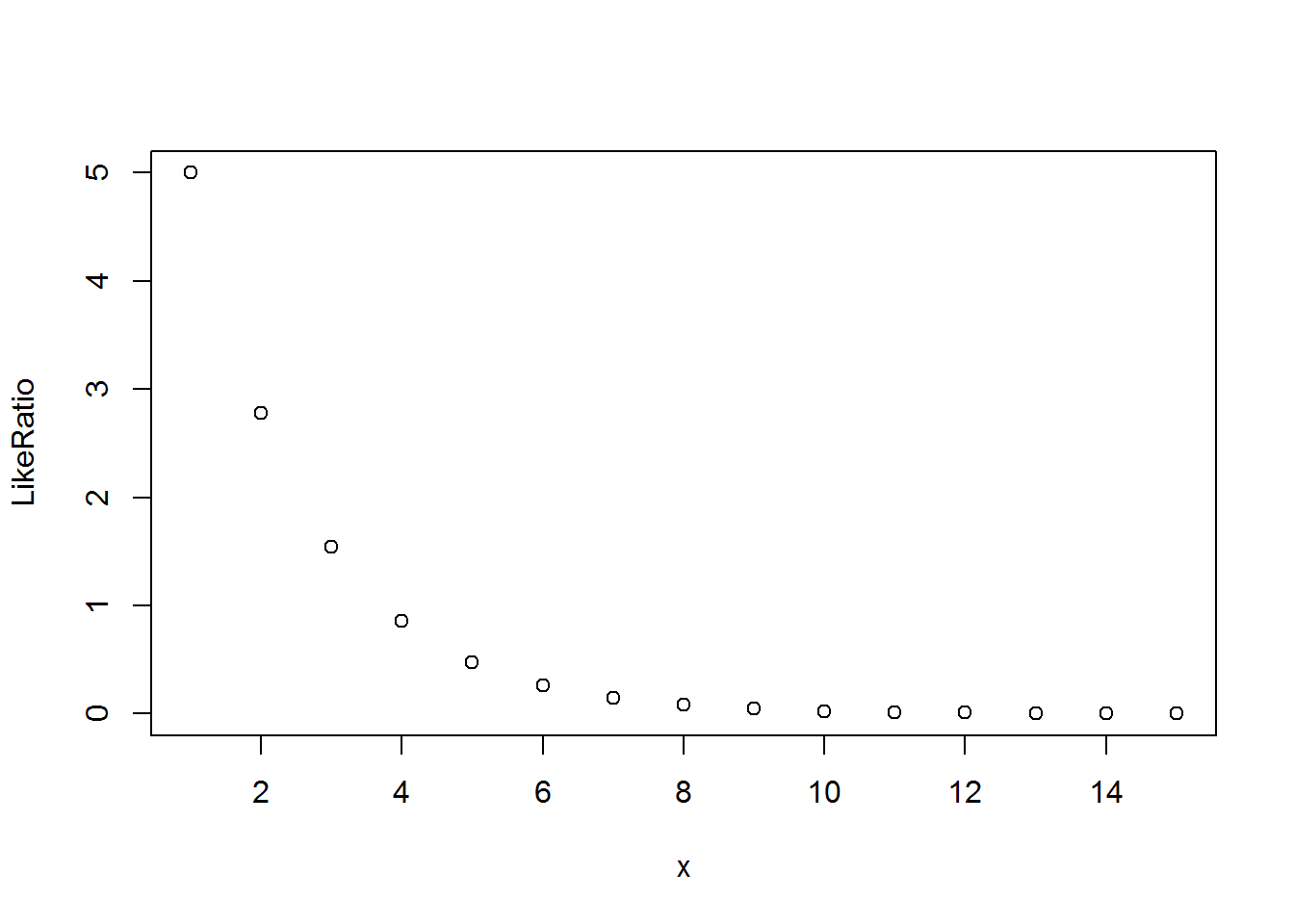# Problem 9.11.1
# A coin is thrown independently 10 times with P(heads)=p
# To test null hypothesis p=.5 versus alternative that it is not,
# reject if 0 or 10 heads observed
#
# Let X be the number of heads observed.
# X ~ Binomial(size=10,prob=p)
#
# a). The significance level of the test is the
#     probability of rejcting the null when it is true.
#     (chance of getting 10 tails or getting 10 heads in a row)
sig.level=2*(.5^10)
print(sig.level)
##  0.001953125
# b). What is the power of the test if P(heads)=.1

# The power is chance of a binomial(size=10,prob=.1) equalling 0 or 10
# This can be computing usin binomial pmf:
dbinom(0,size=10,prob=.1) + dbinom(10,size=10,prob=.1)
##  0.3486784
# Or using the binomial cdf:
pbinom(0,size=10,prob=.1) + (1-pbinom(9,size=10,prob=.1))
##  0.3486784
# Problem 9.11.6.  Consider tossing the coin until a head comes up
#   Define X to be the total number of tosses.
#   The variable Y=(X-1) has a geometric distribution in R
#       with pmf function dgeom(x, prob)

args(dgeom)
## function (x, prob, log = FALSE)
## NULL
x.grid=seq(1,15)
dgeom(0:10,prob=.5)
##   0.5000000000 0.2500000000 0.1250000000 0.0625000000 0.0312500000
##   0.0156250000 0.0078125000 0.0039062500 0.0019531250 0.0009765625
##  0.0004882812
dgeom(0:10,prob=.1)
##   0.10000000 0.09000000 0.08100000 0.07290000 0.06561000 0.05904900
##   0.05314410 0.04782969 0.04304672 0.03874205 0.03486784
x.grid.probs.h0=dgeom(x.grid-1, prob=.5)
x.grid.probs.h1=dgeom(x.grid-1, prob=.1)
x.grid.likeratio=x.grid.probs.h0/x.grid.probs.h1
plot(x.grid, x.grid.likeratio,xlab="x", ylab="LikeRatio")# a). If the prior probabilities are equal, which
#     outcomes favor H0?
#
# These are the values of x for which the likelihood ratio exceeds 1.

x.grid[which(x.grid.likeratio>1)] # x=1,2, or 3
##  1 2 3
# The values which favor H1 are the complement, values greater than 3.

# b). If the prior odds P(H0)/P(H1)=10,
# then the outcomes that favor H0 are those for which the
# posterior odds exceed 1, which are those for which the
# likelihood ratio exceeds 1/10:
x.grid[which(x.grid.likeratio>.1)]
##  1 2 3 4 5 6 7
# c). What is the significance level of a test that
#     rejects H0 if X >= 8
prob.h0=0.5
#     Equals 1-Prob(accept H0 | H0)
sig.level=1-sum(dgeom(c(0:(7-1)), prob=prob.h0))
print(sig.level)
##  0.0078125
# This should be close to
sum(dgeom(7:50,prob=prob.h0))
##  0.0078125
# d) The power of the test is the probability of rejecting
#     given prob=.1

prob.h1=.1
power.h1=1-sum(dgeom(c(0:(7-1)), prob=prob.h1))
print(power.h1)
##  0.4782969
# This should be close to
sum(dgeom(7:50,prob=prob.h1))
##  0.4736585